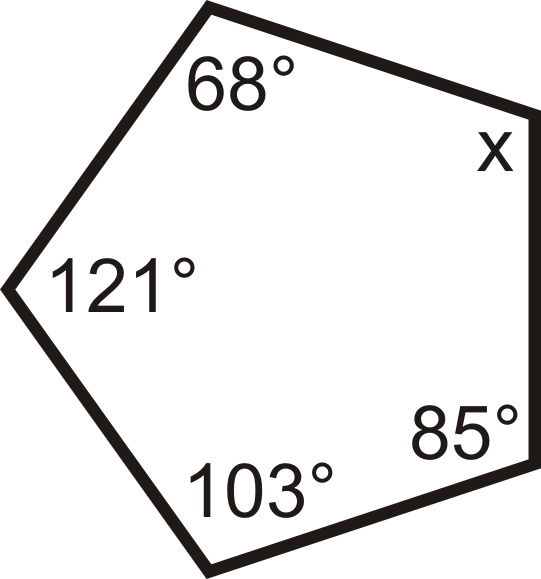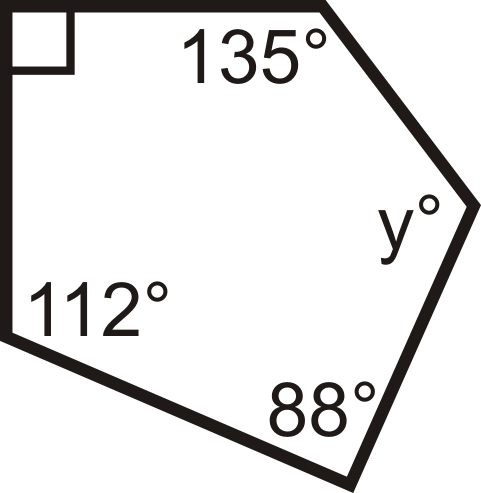# 5.27: Interior Angles in Convex Polygons

•• Contributed by CK12
• CK12

Use the formula $$(x - 2)180$$ to find the sum of the interior angles of any polygon.

The interior angle of a polygon is one of the angles on the inside, as shown in the picture below. A polygon has the same number of interior angles as it does sides.Figure $$\PageIndex{1}$$

The sum of the interior angles in a polygon depends on the number of sides it has. The Polygon Sum Formula states that for any n−gon, the interior angles add up to $$(n−2)\times 180^{\circ}$$.Figure $$\PageIndex{2}$$

\begin{aligned}\rightarrow n=8 \\ (8−2) &\times 180^{\circ} \\ 6 &\times 180^{\circ} \\ &1080^{\circ}\end{aligned}

Once you know the sum of the interior angles in a polygon it is easy to find the measure of ONE interior angle if the polygon is regular: all sides are congruent and all angles are congruent. Just divide the sum of the angles by the number of sides.

Regular Polygon Interior Angle Formula: For any equiangular n−gon, the measure of each angle is $$\dfrac{(n−2)\times 180^{\circ}}{n}$$.Figure $$\PageIndex{3}$$

In the picture below, if all eight angles are congruent then each angle is $$\dfrac{(8−2)\times 180^{\circ}}{8}=\dfrac{6\times 180^{\circ}}{8}=\dfrac{1080^{\circ}}{8}=135^{\circ}$$.Figure $$\PageIndex{4}$$

What if you were given an equiangular seven-sided convex polygon? How could you determine the measure of its interior angles?

Example $$\PageIndex{1}$$

The interior angles of a pentagon are $$x^{\circ}$$, $$x^{\circ}$$, $$2x^{\circ}$$, $$2x^{\circ}$$, and $$2x^{\circ}$$. What is $$x$$?

Solution

From the Polygon Sum Formula we know that a pentagon has interior angles that sum to $$(5−2)\times 180^{\circ}=540^{\circ}$$.

Write an equation and solve for x.

\begin{aligned} x^{\circ}+x^{\circ}+2x^{\circ}+2x^{\circ}+2x^{\circ}&=540^{\circ} \\ 8x&=540 \\ x&=67.5\end{aligned}

Example $$\PageIndex{2}$$

What is the sum of the interior angles in a 100-gon?

Solution

Use the Polygon Sum Formula. $$(100−2)\times 180^{\circ}=17,640^{\circ}$$.

Example $$\PageIndex{3}$$

The interior angles of a polygon add up to $$1980^{\circ}$$. How many sides does it have?

Solution

Use the Polygon Sum Formula and solve for n\).

\begin{aligned} (n−2)\times 180^{\circ}&=1980^{\circ} \\ 180^{\circ}n−360^{\circ}&=1980^{\circ} \\ 180^{\circ}n&=2340^{\circ} \\ n&=13\end{aligned}

The polygon has 13 sides.

Example $$\PageIndex{4}$$

How many degrees does each angle in an equiangular nonagon have?

Solution

First we need to find the sum of the interior angles; set $$n=9$$.

$$(9−2)\times 180^{\circ}=7\times 180^{\circ}=1260^{\circ}$$

“Equiangular” tells us every angle is equal. So, each angle is $$\dfrac{1260^{\circ}}{9}=140^{\circ}$$.

Example $$\PageIndex{5}$$

An interior angle in a regular polygon is $$135^{\circ}$$. How many sides does this polygon have?

Solution

Here, we will set the Regular Polygon Interior Angle Formula equal to $$135^{\circ}$$ and solve for n.

\begin{aligned} \dfrac{(n−2)\times 180^{\circ}}{n}&=135^{\circ} \\ 180^{\circ}n−360^{\circ}−360^{\circ}&=135^{\circ}n \\ n&=−45^{\circ} \\ n&=8\qquad \text{The polygon is an octagon.} \end{aligned}

## Review

1. Fill in the table.
# of sides Sum of the Interior Angles Measure of Each Interior Angle in a Regular n−gon
3 $$60^{\circ}$$
4 $$360^{\circ}$$
5 $$540^{\circ}$$ $$108^{\circ}$$
6 $$120^{\circ}$$
7
8
9
10
11
12
1. What is the sum of the angles in a 15-gon?
2. What is the sum of the angles in a 23-gon?
3. The sum of the interior angles of a polygon is $$4320^{\circ}$$. How many sides does the polygon have?
4. The sum of the interior angles of a polygon is $$3240^{\circ}$$. How many sides does the polygon have?
5. What is the measure of each angle in a regular 16-gon?
6. What is the measure of each angle in an equiangular 24-gon?
7. Each interior angle in a regular polygon is $$156^{\circ}$$. How many sides does it have?
8. Each interior angle in an equiangular polygon is $$90^{\circ}$$. How many sides does it have?

For questions 10-18, find the value of the missing variable(s).

1.Figure $$\PageIndex{5}$$
2.Figure $$\PageIndex{6}$$
3.Figure $$\PageIndex{7}$$
4.Figure $$\PageIndex{8}$$
5.Figure $$\PageIndex{9}$$
6.Figure $$\PageIndex{10}$$
7.Figure $$\PageIndex{11}$$
8.Figure $$\PageIndex{12}$$
9.Figure $$\PageIndex{13}$$
1. The interior angles of a hexagon are $$x^{\circ}$$, $$(x+1)^{\circ}$$, $$(x+2)^{\circ}$$, $$(x+3)^{\circ}$$,$$(x+4)^{\circ}$$, and $$(x+5)^{\circ}$$. What is $$x$$?

## Vocabulary

Term Definition
Interior angles Interior angles are the angles inside a figure.
Polygon Sum Formula The Polygon Sum Formula states that for any polygon with n sides, the interior angles add up to $$(n−2)\times 180$$ degrees.

Interactive Element

Video: Interior and Exterior Angles of a Polygon

Activities: Interior Angles in Convex Polygons Discussion Questions

Study Aids: Polygons Study Guide

Practice: Interior Angles in Convex Polygons

Real World: Interior Angles In Convex Polygons